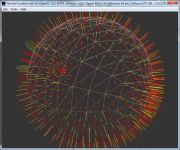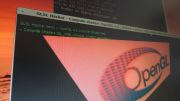Home Utilities Tutorials 3D Demos Graphics Cards Forums About

 GeeXLab
Current version: 0.29.17
>GeeXLab homepage

 FurMark
Current version: 1.21.1
>FurMark homepage

 GPU Caps Viewer
Current version: 1.44.2.1
>GPU Caps Viewer homepage

 GPU Shark
Current version: 0.16.1.0
>GPU Shark homepage

 Blogs
>JeGX's HackLab

 Geeks3D's Articles
>GPU Memory Speed Demystified

>Multi-Threading Programming Resources

>GeForce and Radeon OpenCL Overview

>How to Get your Multi-core CPU Busy at 100%

>How To Make a VGA Dummy Plug

>Night Vision Post Processing Filter

 PhysX FluidMark
Current version: 1.5.4
>FluidMark homepage

 TessMark
Current version: 0.3.0
>TessMark homepage

 ShaderToyMark
Current version: 0.3.0
>ShaderToyMark homepage
>ShaderToyMark Scores

 Demoniak3D
Current Version: 1.23.0
>Demoniak3D
>Download
>Libraries and Plugins
>Demos
>Online Help - Reference Guide
>Codes Samples

3D Graphics Search Engine:Mesh Deformers with the GLSL By Jerome Guinot aka 'JeGX' - jegx_AT_ozone3d(dot)net Initial draft: June 1, 2006 [ Index ]»Next Page 3 - Mesh TwisterLet's tackle chapter 1.3 of the ShaderX3 book and more particularly the part called Twist. The twist effect is cool but the implémentation has been done with Direct3D shaders and above all, the image at the beginning of the chapter showing a nice twisted cube has no implementation. The effect I wish is the following:The twist effect consists in deforming the mesh's vertices along a certain axis. In the demo, the deformation axis is the Y one. In comparison to the surface deformer, the mathematics is less complex and remains limited to few sin/cos cleverly organized functions. The mathematical relation to twist the mesh around the Y axis is the following: new_x = x*cos(angle) - z*sin(angle) new_y = y new_z = x*sin(angle) + z*cos(angle) From this relation and using an angle value based on both time and vertex's position we can achieve the effect shown in the previous image. The objective is to move the vertices with an angle that is proportinal to the elapsed time and the vertex's height. The vertices that have a height equal to 0 will not move while those localized in the upper part of the cube will be deformed with a maximal angle. The following code is that of the vertex shader. The vertex shader's core is the DoTwist() function that is used to tranform a vertex. ```uniform float time; uniform float height; uniform float angle_deg_max; varying vec3 normal, lightDir, eyeVec; vec4 DoTwist( vec4 pos, float t ) { float st = sin(t); float ct = cos(t); vec4 new_pos; new_pos.x = pos.x*ct - pos.z*st; new_pos.z = pos.x*st + pos.z*ct; new_pos.y = pos.y; new_pos.w = pos.w; return( new_pos ); } void main(void) { float angle_deg = angle_deg_max*sin(time); float angle_rad = angle_deg * 3.14159 / 180.0; float ang = (height*0.5 + gl_Vertex.y)/height * angle_rad; vec4 twistedPosition = DoTwist(gl_Vertex, ang); vec4 twistedNormal = DoTwist(vec4(gl_Normal, ang), gl_Position = gl_ModelViewProjectionMatrix * twistedPosition; vec3 vVertex = vec3(gl_ModelViewMatrix * twistedPosition); lightDir = vec3(gl_LightSource.position.xyz - vVertex); lightDir = vec3(gl_LightSource.position.xyz - vVertex); lightDir = vec3(gl_LightSource.position.xyz - vVertex); eyeVec = -vVertex; normal = gl_NormalMatrix * twistedNormal.xyz; gl_TexCoord = gl_MultiTexCoord0; }``` We are in a situation where the vertex normal vector calculation is simple. Indeed, the same function is used to change the vertex's position and to compute its normal. [ Index ]»Next Page

 GeeXLab demosGLSL - Mesh exploderPhysX 3 cloth demoNormal visualizer with GSCompute Shaders test on RadeonRaymarching in GLSL

 Misc
>Texture DataPack #1
>Asus Silent Knight CPU Cooler
 © 2002-2018 oZone3D.Net - G3D Network - All rights reserved. Last update: May 06, 2015 - 08:46
Page generated in 0.0034580230712891 seconds.